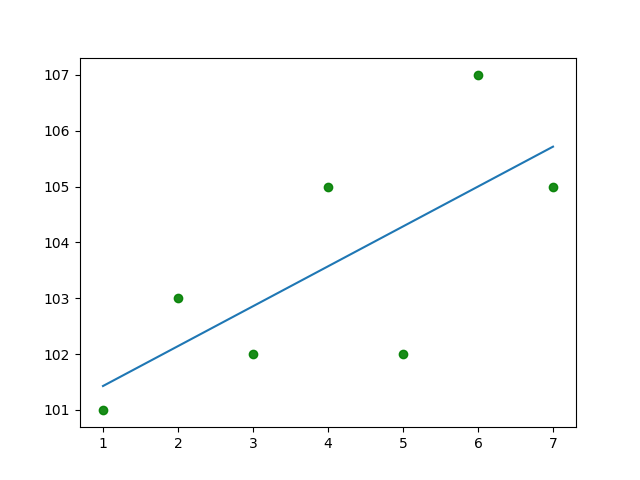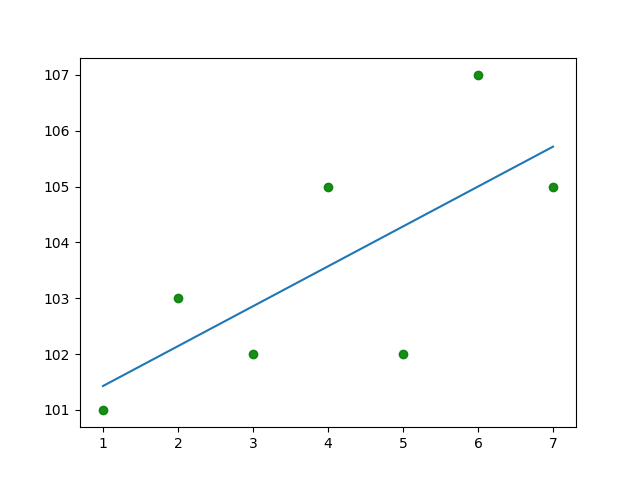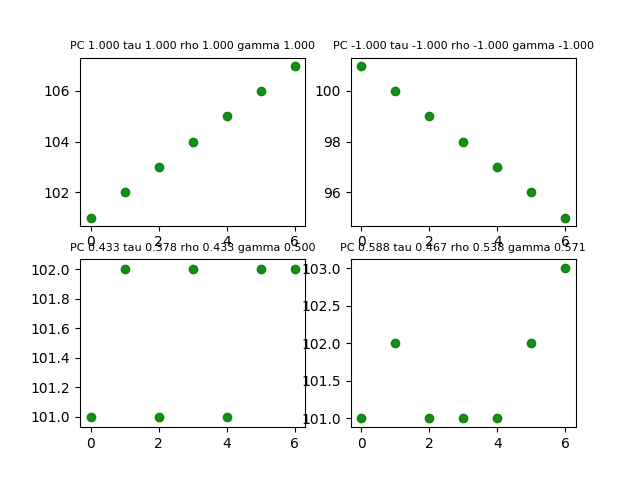## Resources for Learning Statistics

Various online resources (online sources, text books) are freely available on internet.

There are so many online courses that you might get overwhelmed bu the sheer numbers.

## What is Linear Regression

Linear regression attempts to model the relationship between two variables by fitting a linear equation to observed data. One variable is considered to be an explanatory variable, and the other is considered to be a dependent variable. The case of one explanatory variable is called simple linear regression. For more than one explanatory variable, the process is called multiple linear regression.

Linear regression models are often fitted using the least squares approach.

If there appears to be no association between the proposed explanatory and dependent variables (i.e., the scatterplot does not indicate any increasing or decreasing trends), then fitting a linear regression model to the data probably will not provide a useful model. A valuable numerical measure of association between two variables is the correlation coefficient, which is a value between -1 and 1 indicating the strength of the association of the observed data for the two variables.

There are many names for a regression’s dependent variable. It may be called an outcome variable, criterion variable, endogenous variable, or regressand. The independent variables can be called exogenous variables, predictor variables, or regressors.

Linear regression using python

Following are the ways to do linear regression using python

1. statsmodels
2. scikit-learn
3. scipy

### Linear Regression using statsmodels

Here is sample code

 ################################################################################################ # name: linear-regression-01-statsmodels.py # desc: linear regression using statsmodels # date: 2018-07-14 # Author: conquistadorjd # reference: http://www.statsmodels.org/dev/examples/notebooks/generated/ols.html ################################################################################################ import numpy as np import statsmodels.api as sm from scipy import stats import matplotlib.pyplot as plt print('*** Program started ***') ##################################### Testing different patterns y1=[101,102,103,104,105,106,107] y2=[101,100,99,98,97,96,95] y3=[101,102,101,102,101,102,101] y4=[101,103,105,107,109,111,115] y5=[101,103,102,105,102,107,105] y6=[1,2,3,4,5,6,7] y=y5 x =np.arange(len(y)) x = x +1 # this is to preserve original x values to be used for plotting x1=x # This is needed as per statsmodel documentation x=sm.add_constant(x) ##################################### regression model = sm.OLS(y,x) results = model.fit() # print(results.summary()) # print('Parameters: ', results.params) print( 'results.params : ',results.params) # pc = stats.pearsonr(x,y5) # print(pc) # tau = stats.kendalltau(x,y5) # print(tau) # rho = stats.spearmanr(x,y5) # print(rho) # creating regression line xx= x1 yy = results.params + x1*results.params plt.scatter(x1,y,s=None, marker='o',color='g',edgecolors='g',alpha=0.9,label="Jagur") plt.plot(xx,yy) # plt.xlabel('Sample x Axis') # plt.ylabel('Sample y Axis') # plt.legend(loc=2) # plt.grid(color='black', linestyle='-', linewidth=0.5) # plt.title('PC '+ "{:.3f}".format(pc) + ' tau ' + "{:.3f}".format(tau) + ' rho ' + "{:.3f}".format(rho)+ ' gamma ' + "{:.3f}".format(gamma), fontsize=8) # Saving image plt.savefig('linear-regression-01-statsmodels.png') # # In case you dont want to save image but just displya it plt.show() print('*** Program ended ***')

and here is the output### Linear Regression using scikit-learn

Here is the code

 ################################################################################################ # name: linear-regression-02-scikit-learn.py # desc: linear regression using scikit-learn # date: 2018-07-14 # Author: conquistadorjd # reference: http://scikit-learn.org/stable/auto_examples/linear_model/plot_ols.html ################################################################################################ import numpy as np import matplotlib.pyplot as plt from sklearn import datasets, linear_model from sklearn.metrics import mean_squared_error, r2_score from scipy import stats print('*** Program started ***') ##################################### Testing different patterns y1=[101,102,103,104,105,106,107] y2=[101,100,99,98,97,96,95] y3=[101,102,101,102,101,102,101] y4=[101,103,105,107,109,111,115] y5=[101,103,102,105,102,107,105] y=[1,2,3,4,5,6,7] y=y5 x=np.arange(len(y)) x1=np.arange(len(y)) x = x +1 # to ensure count is starting from 1 x = np.array(x).reshape(–1, 1) ##################################### regression regr = linear_model.LinearRegression() regr.fit(x, y) print('Coefficients: \n', regr) m=regr.coef_ b=regr.intercept_ print("slope=",m, "\nintercept=",b) pc = stats.pearsonr(x1,y) print(pc) # tau = stats.kendalltau(x,y) # print(tau) # rho = stats.spearmanr(x,y) # print(rho) xx= x yy = regr.predict(xx) plt.scatter(x,y,s=None, marker='o',color='g',edgecolors='g',alpha=0.9,label="Jagur") plt.plot(xx,yy) # plt.xlabel('Sample x Axis') # plt.ylabel('Sample y Axis') # plt.legend(loc=2) # plt.grid(color='black', linestyle='-', linewidth=0.5) # plt.title('PC '+ "{:.3f}".format(pc) + ' tau ' + "{:.3f}".format(tau) + ' rho ' + "{:.3f}".format(rho)+ ' gamma ' + "{:.3f}".format(gamma), fontsize=8) # Saving image plt.savefig('linear-regression-02-scikit-learn.png') # In case you dont want to save image but just displya it plt.show() print('*** Program ended ***')

and output of this code is as below### Linear Regression using scipy

Sample code

 ################################################################################################ # name: linear-regression-03-scipy.py # desc: linear regression using scipy # date: 2018-07-14 # Author: conquistadorjd # reference: https://docs.scipy.org/doc/scipy/reference/generated/scipy.stats.linregress.html ################################################################################################ import numpy as np import matplotlib.pyplot as plt from sklearn import datasets, linear_model from sklearn.metrics import mean_squared_error, r2_score from scipy import stats print('*** Program started ***') ##################################### Testing different patterns y1=[101,102,103,104,105,106,107] y2=[101,100,99,98,97,96,95] y3=[101,102,101,102,101,102,101] y4=[101,103,105,107,109,111,115] y5=[101,103,102,105,102,107,105] y6=[1,2,3,4,5,6,7] y=y5 x=np.arange(len(y)) x1=np.arange(len(y)) x = x +1 # to ensure count is starting from 1 ##################################### regression slope, intercept, r_value, p_value, std_err = stats.linregress(x, y) print('Coefficients: \n', slope, intercept, r_value, p_value, std_err) pc = stats.pearsonr(x1,y) print(pc) # tau = stats.kendalltau(x,y) # print(tau) # rho = stats.spearmanr(x,y) # print(rho) # xx= x # yy = regr.predict(xx) plt.scatter(x,y,s=None, marker='o',color='g',edgecolors='g',alpha=0.9,label="Jagur") plt.plot(x, intercept + slope*x, label='fitted line') # # plt.xlabel('Sample x Axis') # # plt.ylabel('Sample y Axis') # # plt.legend(loc=2) # # plt.grid(color='black', linestyle='-', linewidth=0.5) # # plt.title('PC '+ "{:.3f}".format(pc) + ' tau ' + "{:.3f}".format(tau) + ' rho ' + "{:.3f}".format(rho)+ ' gamma ' + "{:.3f}".format(gamma), fontsize=8) # # Saving image plt.savefig('linear-regression-03-scipy.png') # # In case you dont want to save image but just displya it plt.show() print('*** Program ended ***')

and outputIf you look at code, it seems finding linear regression using scipy is shortest and easiest to understand.

## What is Correlation ?

Correlation is  used to indicate dependence or association is any statistical relationship, whether causal or not, between two random variables or bivariate data. It is a measure of relationship between two mathematical variables or measured data values, which includes the Pearson correlation coefficient as a special case.Correlation is any of a broad class of statistical relationships involving dependence, though in common usage it most often refers to how close two variables are to having a linear relationship with each other.

The strength of the linear association between two variables is quantified by the correlation coefficient.

Formula for correlation is as below

• The correlation coefficient always takes a value between -1 and 1,
• Value of 1 or -1 indicating perfect correlation (all points would lie along a straight line in this case).
• A correlation value close to 0 indicates no association between the variables.The closer the value of r to 0 the greater the variation around the line of best fit.
• A positive correlation indicates a positive association between the variables (increasing values in one variable correspond to increasing values in the other variable),
• while a negative correlation indicates a negative association between the variables (increasing values is one variable correspond to decreasing values in the other variable).

The square of the correlation coefficient, r², is a useful value in linear regression. This value represents the fraction of the variation in one variable that may be explained by the other variable. Thus, if a correlation of 0.8 is observed between two variables (say, height and weight, for example), then a linear regression model attempting to explain either variable in terms of the other variable will account for 64% of the variability in the data1

the least-squares regression line will always pass through the means of x and y, the regression line may be entirely described by the means, standard deviations, and correlation of the two variables under investigation.

## Pearson correlation coefficient

Pearsons correlation coefficient is a measure of the linear correlation between two variables X and Y. It has a value between +1 and −1 2.t is obtained by dividing the covariance of the two variables by the product of their standard deviations.

Formula for Pearson Correlation Coefficient## Rank correlation coefficients

### Spearman’s rank correlation coefficient

The Spearman correlation coefficient is defined as the Pearson correlation coefficient between the ranked variables.3

### Kendall rank correlation coefficient

the Kendall correlation between two variables will be high when observations have a similar (or identical for a correlation of 1) rank (i.e. relative position label of the observations within the variable: 1st, 2nd, 3rd, etc.) between the two variables, and low when observations have a dissimilar (or fully different for a correlation of -1) rank between the two variables.4

## Goodman and Kruskal’s gamma

Goodman and Kruskal’s gamma is a measure of rank correlation, i.e., the similarity of the orderings of the data when ranked by each of the quantities. 5

You can find his report here

Now let us try to calculate these correlations using python, you can find code below

 ################################################################################################ # name: correlationexamples-00.py # desc: Correlations # date: 2018-07-14 # Author: conquistadorjd # remark : goodman_kruskal_gamma formula taken from https://github.com/shilad/context-sensitive-sr/blob/master/SRSurvey/src/python/correlation.py ################################################################################################ from matplotlib import pyplot as plt import numpy as np from scipy import stats from itertools import combinations, permutations def goodman_kruskal_gamma(m, n): """ compute the Goodman and Kruskal gamma rank correlation coefficient; this statistic ignores ties is unsuitable when the number of ties in the data is high. it's also slow. >>> x = [2, 8, 5, 4, 2, 6, 1, 4, 5, 7, 4] >>> y = [3, 9, 4, 3, 1, 7, 2, 5, 6, 8, 3] >>> goodman_kruskal_gamma(x, y) 0.9166666666666666 """ num = 0 den = 0 for (i, j) in permutations(range(len(m)), 2): m_dir = m[i] – m[j] n_dir = n[i] – n[j] sign = m_dir * n_dir if sign > 0: num += 1 den += 1 elif sign < 0: num -= 1 den += 1 return num / float(den) print('*** Program Started ***') # x=[1,2,3,4,5] y1=[101,102,103,104,105,106,107] y2=[101,100,99,98,97,96,95] y3=[101,102,101,102,101,102,102] y4=[101,102,101,101,101,102,103] # y3=y2 # y4=y1 x=np.arange(len(y1)) pc = stats.pearsonr(x,y1) tau = stats.kendalltau(x,y1) rho = stats.spearmanr(x,y1) gamma = goodman_kruskal_gamma(x,y1) ax1 = plt.subplot(221) plt.scatter(x,y1,s=None, marker='o',color='g',edgecolors='g',alpha=0.9,label="Jagur") # plt.xlabel('Sample x Axis') # plt.ylabel('Sample y Axis') # plt.legend(loc=2) # plt.grid(color='black', linestyle='-', linewidth=0.5) plt.title('PC '+ "{:.3f}".format(pc) + ' tau ' + "{:.3f}".format(tau) + ' rho ' + "{:.3f}".format(rho)+ ' gamma ' + "{:.3f}".format(gamma)) pc = stats.pearsonr(x,y2) tau = stats.kendalltau(x,y2) rho = stats.spearmanr(x,y2) gamma = goodman_kruskal_gamma(x,y2) ax2 = plt.subplot(222) plt.scatter(x,y2,s=None, marker='o',color='g',edgecolors='g',alpha=0.9,label="Jagur") # plt.xlabel('Sample x Axis') # plt.ylabel('Sample y Axis') # plt.legend(loc=2) # plt.grid(color='black', linestyle='-', linewidth=0.5) plt.title('PC '+ "{:.3f}".format(pc) + ' tau ' + "{:.3f}".format(tau) + ' rho ' + "{:.3f}".format(rho)+ ' gamma ' + "{:.3f}".format(gamma)) pc = stats.pearsonr(x,y3) tau = stats.kendalltau(x,y3) rho = stats.spearmanr(x,y3) gamma = goodman_kruskal_gamma(x,y3) ax2 = plt.subplot(223) plt.scatter(x,y3,s=None, marker='o',color='g',edgecolors='g',alpha=0.9,label="Jagur") # plt.xlabel('Sample x Axis') # plt.ylabel('Sample y Axis') # plt.legend(loc=2) # plt.grid(color='black', linestyle='-', linewidth=0.5) plt.title('PC '+ "{:.3f}".format(pc) + ' tau ' + "{:.3f}".format(tau) + ' rho ' + "{:.3f}".format(rho)+ ' gamma ' + "{:.3f}".format(gamma)) pc = stats.pearsonr(x,y4) tau = stats.kendalltau(x,y4) rho = stats.spearmanr(x,y4) gamma = goodman_kruskal_gamma(x,y4) ax2 = plt.subplot(224) plt.scatter(x,y4,s=None, marker='o',color='g',edgecolors='g',alpha=0.9,label="Jagur") # plt.xlabel('Sample x Axis') # plt.ylabel('Sample y Axis') # plt.legend(loc=2) # plt.grid(color='black', linestyle='-', linewidth=0.5) plt.title('PC '+ "{:.3f}".format(pc) + ' tau ' + "{:.3f}".format(tau) + ' rho ' + "{:.3f}".format(rho)+ ' gamma ' + "{:.3f}".format(gamma)) # Saving image plt.savefig('correlationexamples-01.png') # In case you dont want to save image but just displya it plt.show() print('*** Program ended ***')

output is as below:2.
Pearson_correlation_coefficient. wikipedia. https://en.wikipedia.org. Accessed July 14, 2018.
3.
Spearman’s rank correlation coefficient. wikipedia. https://en.wikipedia.org. Accessed July 14, 2018.
4.
Kendall_rank_correlation_coefficient. wikipedia. https://en.wikipedia.org/. Accessed July 14, 2018.
5.
Goodman and Kruskal’s gamma. wikipedia. https://en.wikipedia.org/wiki/Goodman_and_Kruskal%27s_gamma. Accessed July 14, 2018.

## What is Regression and Types of Regression

2Regression is a set of statistical processes for estimating the relationships among variables. It includes many techniques for modeling and analyzing several variables, when the focus is on the relationship between a dependent variable (target) and one or more independent variables (or ‘predictors’).

Regression analysis helps one understand how the typical value of the dependent variable (or ‘criterion variable’) changes when any one of the independent variables is varied, while the other independent variables are held fixed.

### Usage

It is used in variety of places such as forecasting, time series analysis etc. across industries.

• Regression analysis is used characterize the variation of the dependent variable around the prediction of the regression function using a probability distribution
• A function of the independent variables called the regression function is to be estimated
• Regression analysis can be used to infer causal relationships between the independent and dependent variables. However this can lead to illusions or false relationships, so caution is advisable as correlation does not prove causation.

## Types of regression:

1. Linear Regression
1. Simple Linear Regression
2. multiple linear regression.
2. Logistic Regression
1. Simple Logistic Regression
2. Multiple Logistic Regression
3. Polynomial Regression
4. Stepwise Regression
5. Ridge Regression
6. Lasso Regression
7. ElasticNet Regression1
1.
Ray S. 7 Types of Regression Techniques you should know! analyticsvidhya. https://www.analyticsvidhya.com. Accessed July 14, 2018.
2.
Regression_analysis. wikipedia. https://en.wikipedia.org/wiki/Regression_analysis. Accessed July 14, 2018.

## Getting started with Statistics

Getting started with statistics